# 强大的JS位运算## 为什么是二进制？

$（4231)_{10} = 4*10^3+2*10^2+3*10^1+1*10^0$

$(25)_{10} = 16+8+1 = 1*2^4+1*2^3+0*2^2+0*2^1+1*2^0 =(11001)_2\\$

## 原码，反码，补码$-7 = (-3)*3+2$

$(-7) \mod 3 = 3 - (7\mod 3)$

1. 指针 向前拨 6 个小时，即 $2+6=8$
2. 指针 向后拨 6 个小时，即 $2-6=8$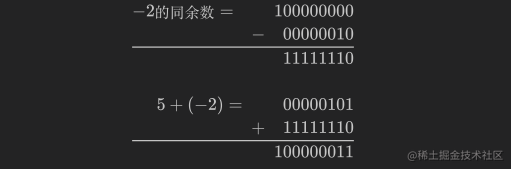-5 的原码：$(10000101)_2$

-5 的反码：$(11111010)_2$

-5 的补码：$(11111011)_2$

### 为什么 c++ 中 int 的范围是 $-2^{31}\ldots(2^{31}-1)$?

+0$(00000000)_2$$(00000000)_2$
-0$(10000000)_2$$(11111111)_2$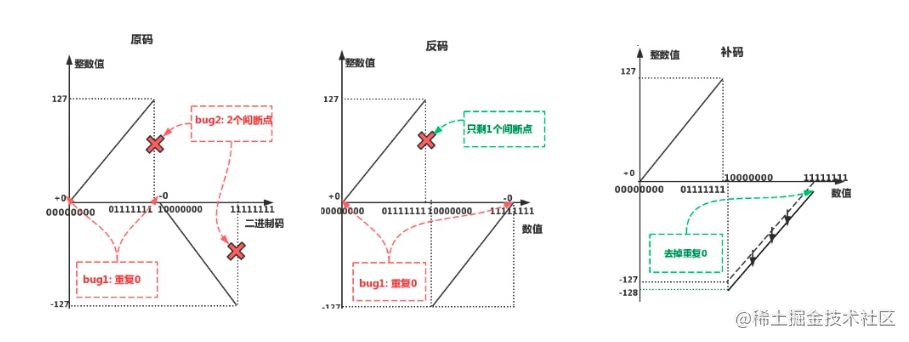c++ int 占用 4 个字节，即 32 位，除去最高位的符号位，还有 31 位，那么正数的所有情况是 $2^{31}$ 种，正数是从 0 开始，所以正数的范围是 $0\ldots2^{31}-1$，而负数这边同样是 $2^{31}$ 种，但是由于人为指定 $-0$ 被人为的指定为 $-2^{31}$，所以负数的范围是 $(-1)\ldots(-2^{31})$ ，合在一起的范围就是 $-2^{31}\ldots(2^{31}-1)$

## Why 位运算？

console.time("常规判断");
for (let i = 0; i < 100000; i++) {
i % 2 === 0;
}
console.timeEnd("常规判断"); // 10.842ms

console.time("位运算判断");
for (let i = 0; i < 100000; i++) {
(i & 1) === 0;
}
console.timeEnd("位运算判断"); // 0.968ms

## JS 位运算

JS 不区分浮点数和整数，所有数值都以 IEEE-754 64 位格式来存储，但位操作符并不直接操作 64 位的值，而是先将 64 位的值转换为 32 位的整数，再执行操作，最后再将结果转换为 64 位。因此过大过小的数，浮点数会出现精度损失，需要特别注意。

### 与（And &）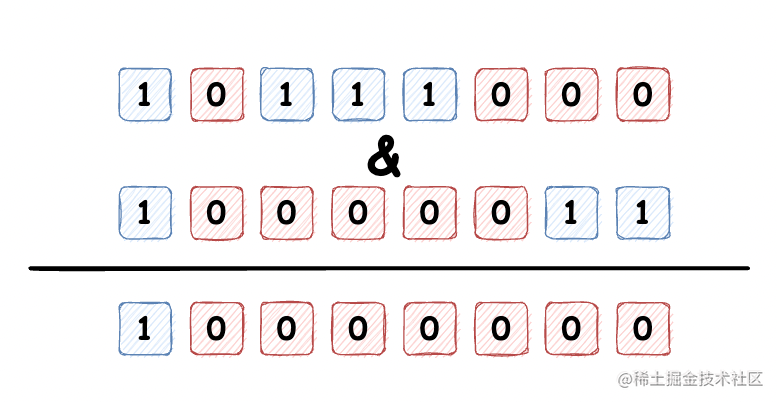### 或（OR |）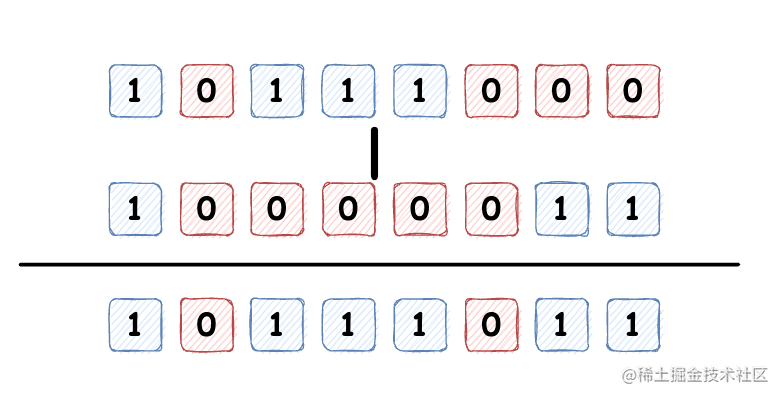### 异或（XOR ^）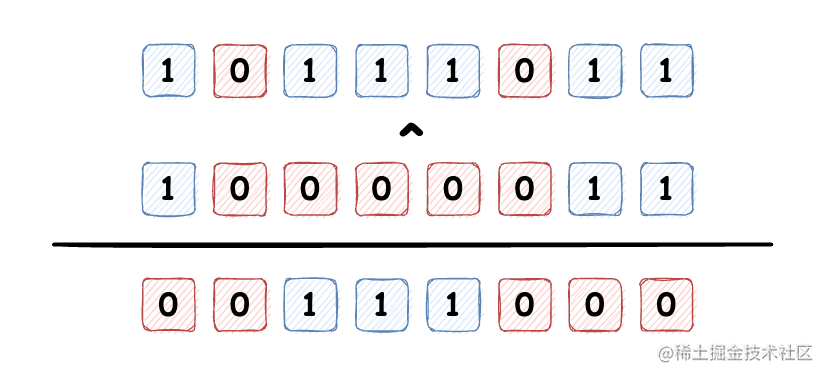• 归零律，即一个数和自身的异或结果总是等于 0，$a \oplus a = 0$
• 恒等律，即一个数与 0 的异或结果总是等于其本身，$a \oplus 0 = a$
• 交换律，即$a \oplus b = b \oplus a$
• 结合律，即$a \oplus (b \oplus c)=(a \oplus b) \oplus c$
• 自反，结合归零律和恒等律，可得出$a \oplus b \oplus a = b$
• 对于任意整数 $i$ ，有 $4i\oplus(4i+1)\oplus(4i+2)\oplus(4i+3)= 0$

let a = 10;
let b = 20;

a = a ^ b;
b = b ^ a;
a = a ^ b;

console.log(a, b); // a = 20, b = 10

### 非（NOT ~）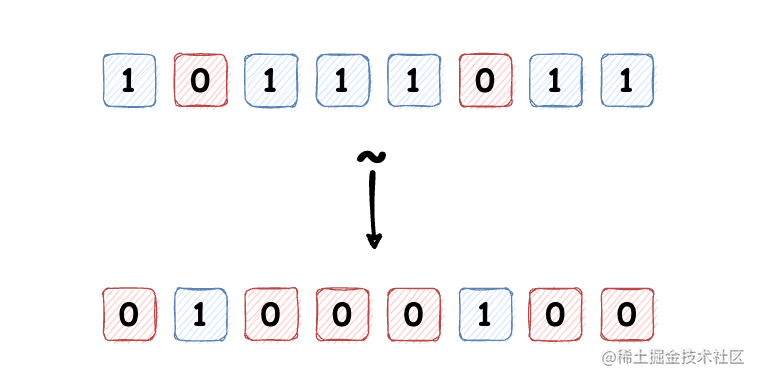const a = [1, 2, 3, 4];
const idx = a.findIndex((i) => i === 2);
// 通常的写法
// if(idx!== 1){
//
// }
// 非运算
if (~idx) {
}

### 向左移位（<<）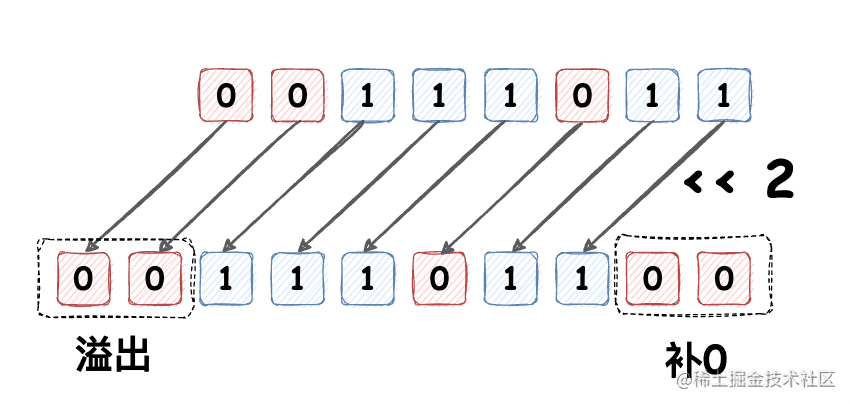### 无符号向右移位（>>>）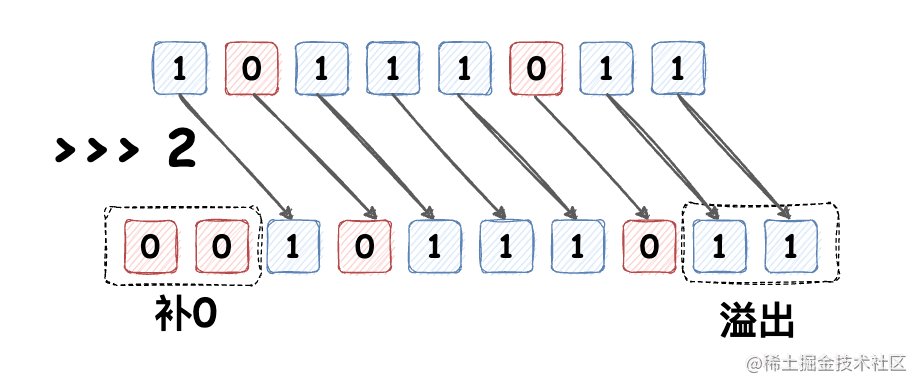### 有符号向右移位（>>）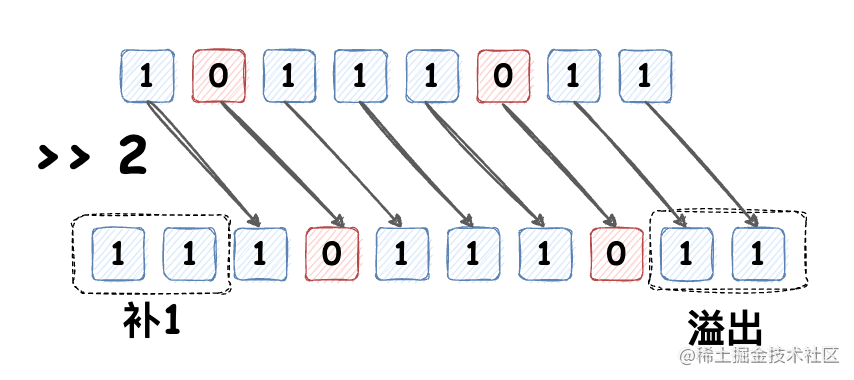stackoverflow 上 What is the JavaScript >>> operator and how do you use it? 也有更详细的解释，也可以查看 ECMA ApplyStringOrNumericBinaryOperator 规范 查看详细的转换流程

### 花式取整

• $234.234 \mid 0 = 234$
• $234.234 \oplus 0 = 234$
• $234.234 >>> 0 = 234$
• $234.234 << 0 = 234$

## 框架中的位运算实践

### React18

React 也使用了在很多场景中使用了位运算进行性能和代码上的优化，比如在组件调用 setState 等方法更新状态后，内部会根据优先级执行更新操作，而这些更新优先级使用了二进制的掩码做处理。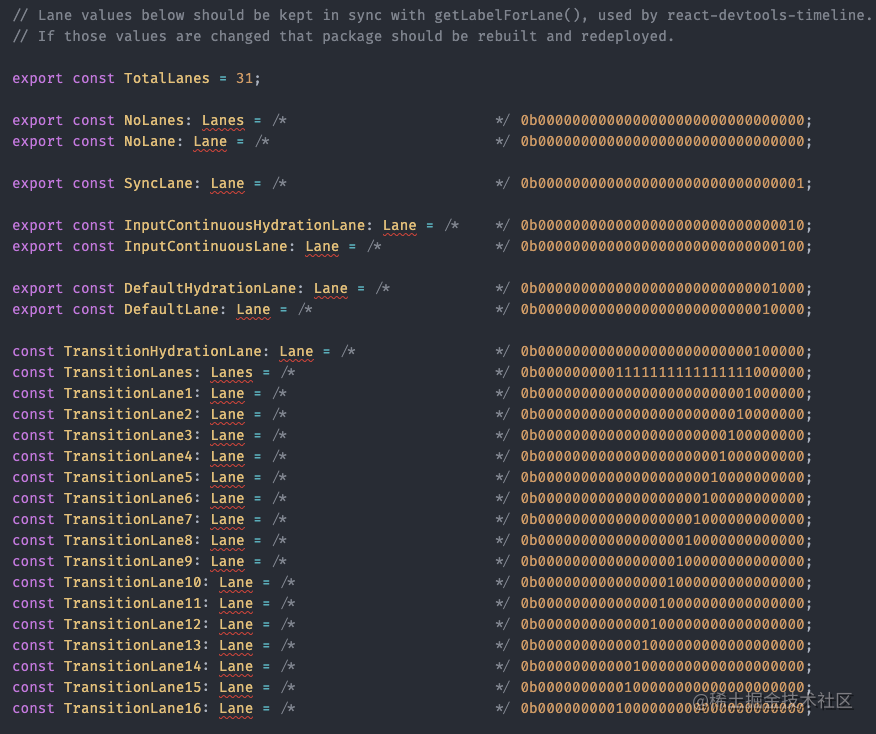Lanes 使用了 31 位长度的二进制码表示优先级（上文已经说过，最高位 32 位是符号位），且 1 的位置越低（即数值越小）表示优先级越高。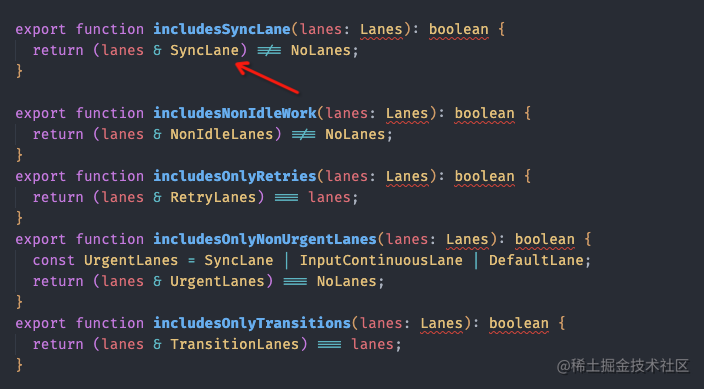React 的更新策略是优先处理高优的更新，所有需要从 wipLane 中挑出最高等级的更新，会用到如下的方法：

export function getHighestPriorityLane(lanes: Lanes): Lane {
return lanes & -lanes;
}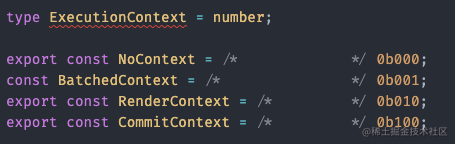### Vue3

Vue3 在对模板代码进行编译时，可以推断出当前节点有哪些动态绑定的属性。在生成代码时，Vue 在 vnode 创建调用中直接编码了每个元素所需的更新类型。而后在运行时，渲染器也会使用位运算操作来检查这些标记，确定相应的更新操作，提高 Diff 性能。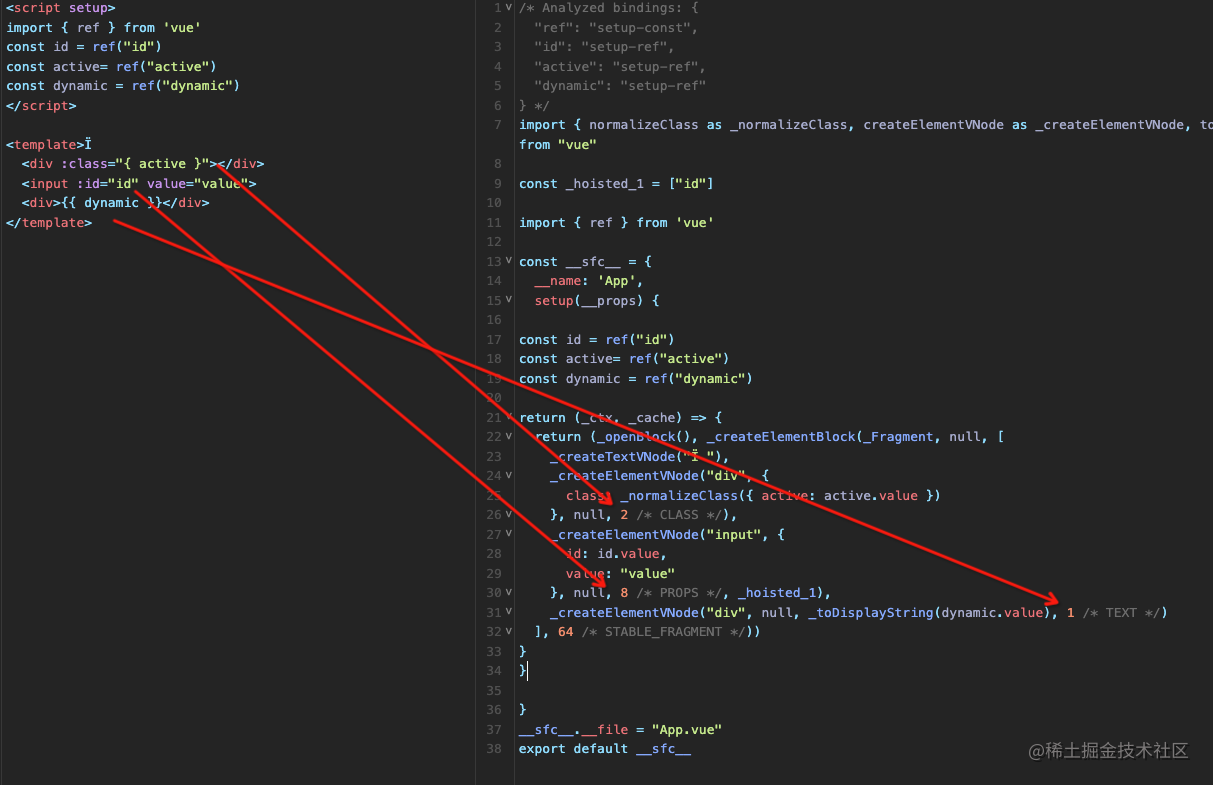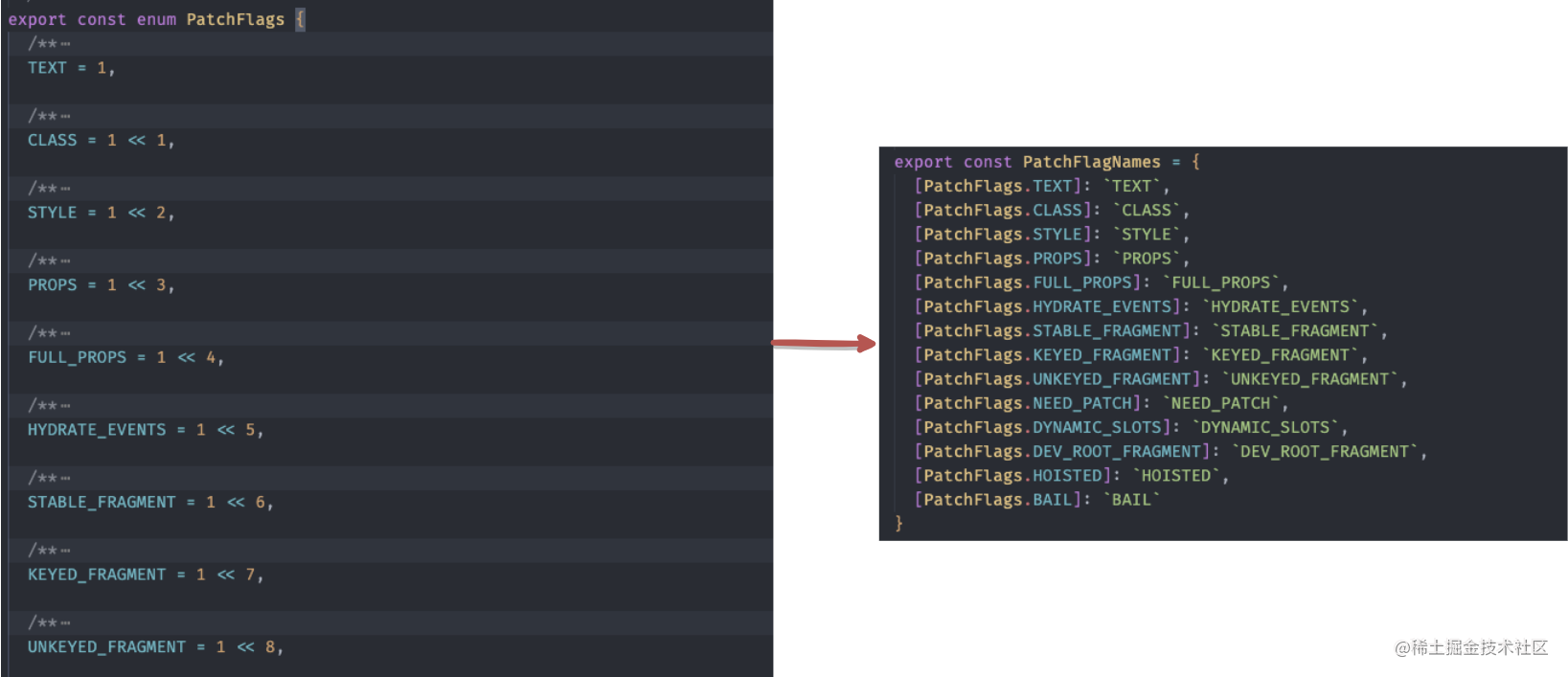patchFlag 的各种操作也不外乎是上面几种位运算的组合。